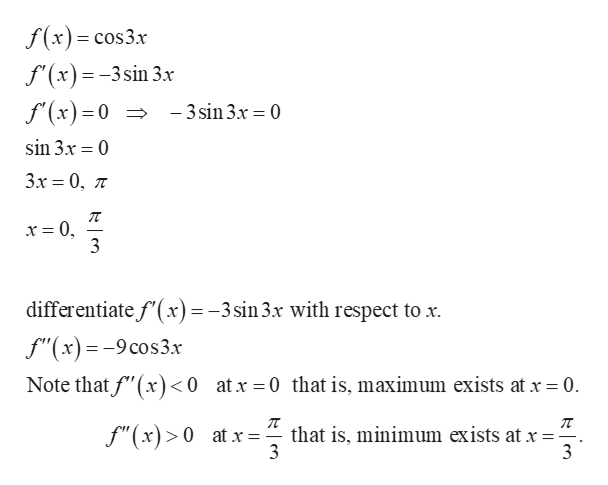# Determine the location and value of the absolute extreme values of f on the given interval, if they exist.f(x)=cos3x on [-pi/4 , pi/2]what is/are the absolute maximum/maxima of f on the given interval?what is/are the absolute mininum/minima of f on the given interval?

Question

Determine the location and value of the absolute extreme values of f on the given interval, if they exist.

f(x)=cos3x on [-pi/4 , pi/2]

what is/are the absolute maximum/maxima of f on the given interval?

what is/are the absolute mininum/minima of f on the given interval?

check_circleExpert Solution
Step 1

Compute as follows.

...help_outlineImage Transcriptionclosef(x)= cos3x f'(x)=-3sin 3x f'(x)=0 >-3sin3x = 0 sin 3.x 0 Зх 3 0, л - x = 0, 3 differentiate f(x) = -3sin3x with respect to x f"(x)=-9cos3x Note that f"(x) <0 atx 0 that is, maximum exists at x 0 that is, minimum exists at x = 3 f"(x)>0 at x 3 fullscreen

### Want to see the full answer?

See Solution

#### Want to see this answer and more?

Solutions are written by subject experts who are available 24/7. Questions are typically answered within 1 hour*

See Solution
*Response times may vary by subject and question
Tagged in

### Other